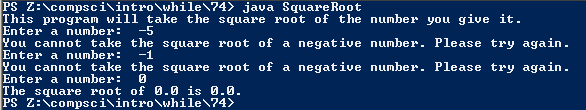# Assignment 74; SquareRoot

## Code

```  // Donovan Rich
// Period 6
// Safe Square Root
// SquareRoot.java
// 12/15/2015

import java.util.Scanner;

public class SquareRoot
{
public static void main( String[] args )
{
Scanner keyboard = new Scanner(System.in);

double num, sq;
System.out.println( "This program will take the square root of the number you give it." );
do
{
System.out.print( "Enter a number:  " );
num = keyboard.nextDouble();
if ( num < 0 )
System.out.println( "You cannot take the square root of a negative number. Please try again." );
} while ( num < 0 );

System.out.println("The square root of " + num + " is " + Math.sqrt(num) + "." );
}
}

```

### Picture of the output